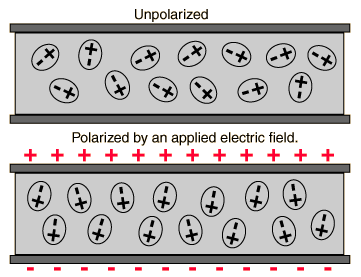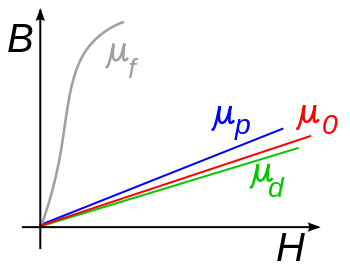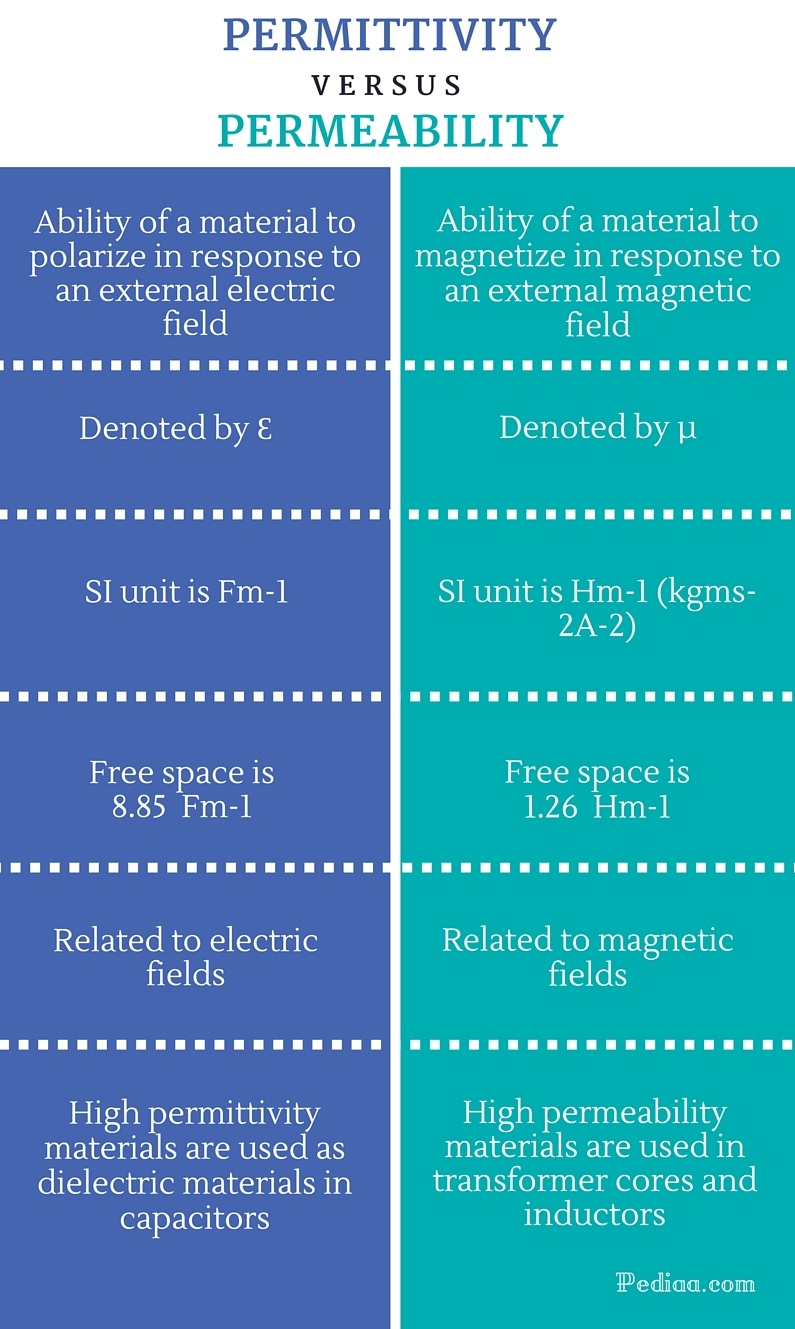# Difference Between Permittivity and Permeability

## Main Difference – Permittivity vs Permeability

Permittivity and permeability are two different measures used in electromagnetism. Permittivity measures the ability of a material to store energy within the material. Permeability, on the other hand, is a measure of the ability of a material to support the formation of a magnetic field within the material. The permittivity of a material is related to the polarization of the material whereas the permeability of a material related to the magnetization of the material.  This is the main difference between permittivity and permeability. Permittivity and permeability have very different and particular meanings in electromagnetism. This article tries to explain them in detail.

## What is Permittivity

The permittivity of material is a measure of the capability of the material to support the formation of an electric field within the material in response to an external electric field. It is commonly denoted by the symbol ε.

The permittivity of free space, also known as vacuum permittivity or electric constant, is usually denoted by the symbol ε0. Its value is 8.85 10-12 Fm-1.

The permittivity of a homogeneous isotropic material is equal to the ratio of the electric displacement field to the electric field. It can be expressed as ε=D/E . where D is the electric displacement field. The permittivity of a material depends on several factors such as frequency of the applied electric field, temperature, humidity and strength of the applied electric field. It has a complex relationship with the frequency of the applied electric field. The static permittivity of a material is a special case, which is the permittivity of a material under the influence of a static electric field.

Usually, the permittivity of a material is expressed as a relative permittivity, which is a dimensionless quantity. Relative permittivity, also known as the dielectric constant, is the ratio of the absolute permittivity of a material to the vacuum permittivity. This relationship can be expressed as εr = ε/ ε0 . Where εr is the relative permittivity of the material. So, the relative permittivity of free space is equal to 1.

Permittivity is a very important quantity in electromagnetism. Usually, the materials having higher values of permittivity are highly polarizable. The higher the permittivity of a medium, the more energy is stored in the medium. So, high permittivity materials are used as dielectric materials in capacitors## What is Permeability

In electromagnetism, the magnetic permeability of a material is a measure of the capability of the material to support the formation of a magnetic field within the material in response to an external magnetic field. In general, the permeability of a material depends on several factors such as the temperature, magnetic field strength, humidity and the frequency of the magnetic field.

The permeability of a material is usually denoted by the symbol µ and is equal to the ratio of the magnetic flux density to the magnetic field strength. It can be expressed as  µ = B/H.

The permeability of free space, also known as the permeability constant, vacuum permeability or magnetic constant of free space, is usually denoted by the symbol μ0. Its value is 4π 10-7 Hm-1.

The ratio of the permeability of a given medium to the permeability of free space is known as relative permeability. So, the relative permeability of a medium is a dimensionless quantity and can be expressed as μr = µ/μ0. According to this definition, the relative permeability of free space is 1. Usually, the permeability of a material is expressed as a relative permeability. The relative permeability of a paramagnetic material is slightly higher than 1. The relative permeability of a diamagnetic material, on the other hand, is slightly less than 1. There is another type of magnetic materials called ferromagnetic materials. The relative permeability of a ferromagnetic material is notably higher than 1. The permeability is a very important quantity especially in material sciences and engineering. For example, it is important to choose a material with high magnetic permeability when designing transformer cores and inductors.## Difference Between Permittivity and Permeability

### Physical Meaning:

Permittivity: Permittivity is the ability of a material to polarize in response to an external electric field.

Permeability: Permeability is the ability of a material to magnetize in response to an external magnetic field.

### Denoted By:

Permittivity: It is denoted by Ԑ.

Permeability: It is denoted by µ.

### SI Unit:

Permittivity: Its SI unit is Fm-1

Permeability: Its SI unit is Hm-1 (kgms-2A-2)

### Value in free space:

Permittivity: Permittivity in free space is 8.85  Fm-1

Permeability: Permeability in free space is 1.26  Hm-1

### Related to:

Permittivity: It is related to electric fields.

Permeability: It is related to magnetic fields.

### Importance of the quantity:

Permittivity: High permittivity materials are used as dielectric materials in capacitors.

Permeability: High permeability materials are used in transformer cores and inductors.Image Courtesy:

“Image 1” by hyperphysics – (Public Domain) via Commons Wikimedia

“Image 2” by Zureks – Own work (Public Domain) via Commons Wikimedia